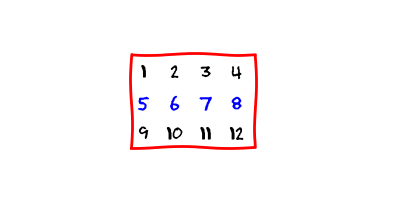The critical construct takes care that a program executes the associated block of code by a single thread at a time. It is not possible that multiple threads execute this block of code at the same time.

The syntax of the critical construct is

In this article, we use the critical construct to avoid data races.

## Has a matrix a zero entry?

We should figure out if a given matrix has a zero entry. For all non-mathematicians out there, a matrix is just a rectangular array of numbers.Each number is one entry. And we should determine if one of the entries is equal to zero.

We write a simple program. The program first initializes a boolean variable `has_zero` to `false`. Then the program loops through each entry and sets the boolean variable to `true` if the entry is equal to zero.

If, in the end, `has_zero` is equal to `true`, then the matrix has a zero entry. If `has_zero` is equal to `false`, then the matrix does not have any zero entries.

Ok, this was not a hard problem. But what if the number of entries of the matrix is very high. We might benefit from a parallel execution of the program. Therefore, let us parallelize it.

## Parallelization

We decide to parallelize the outer loop. We identify all the shared variables in the parallel region: `rows`, `cols`, `matrix` and `has_zero`.

The three variables `rows`, `cols` and `matrix` are read-only variables. They do not cause any problems.

On the other hand, the program modifies `has_zero`. This means that multiple threads might modify this variable at the same time. Here is a potential data race. We must “critically” do something about it!

## It is critical!

OpenMP provides some synchronization mechanisms, which can help us to resolve data races. The critical construct is one of them. It takes care that only one tread executes selected section of code. It is not possible that multiple treads execute this section at the same time.

This is exactly what we need. If only one thread at a time modifies `has_zero`, then there is no data race.

The parallelized version of the program now looks like this:

## Validation

We implement both versions of the program and compare the execution time for a matrix of size `1000 x 1000`. You can access the source code here and play with it.

There is some benefit in the parallel version since its execution time is lower than the time of the sequential version.

Ok, I know. You are getting angry and you think: “What an idiot! He should use the break statement!” Yes, this is a valid concern. Using the break statement in the sequential version would definitely improve the performance. It is also possible to parallelize such a loop.

We will address these issues in the next article ;-).

## Summary

We parallelized a program which checks if a matrix has a zero entry. We used the critical construct to ensure that the program is data race free.

In the next article, we will improve the current program by using the break statement.